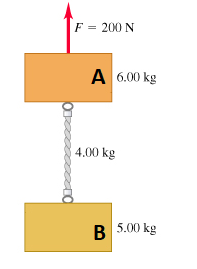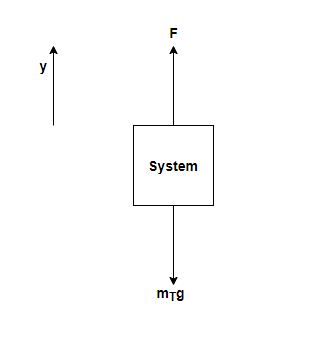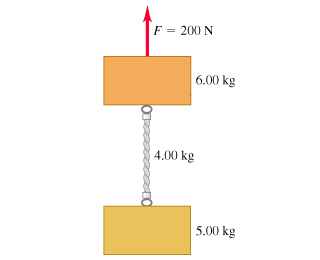# Problem: The two blocks in the figure  are connected by a heavy uniform rope with a mass of 4.00 kg . An upward force of 200 N is applied as shown.(a) What is the acceleration of the system?(b) What is the tension at the top of the heavy rope?(c) What is the tension at the midpoint of the rope?

###### FREE Expert Solution

This problem requires us to determine the system's acceleration and tension at the top and at the middle of the rope.

This is a System of Objects type of problem with Vertical Rope-Connected Objects. Whenever we have such kind of a problem, the steps are simple and straightforward:

1. Draw Free-Body Diagrams (FBDs) for each object in question.
2. Set up Newton's Second Law (ΣF = ma). Note that acceleration will be the same for all the objects.
3. Solve for all the target variables.

For the purpose of consistency, we'll relabel the blocks as follows:We'll draw different free-body diagrams depending on the parts of the question. We'll also consider the motion to be in the y-direction

Each part of the problem will have its own FBD. Thus, we'll use all the steps for each part (a), (b), and (c)

(a) This part asks us to determine the acceleration of the system.

Step 1: Draw FBD for the whole SystemThe forces acting on the system are: Upward force and downward force mTg, where mT is the sum of all masses in the system.

96% (60 ratings)###### Problem Details

The two blocks in the figure  are connected by a heavy uniform rope with a mass of 4.00 kg . An upward force of 200 N is applied as shown.
(a) What is the acceleration of the system?
(b) What is the tension at the top of the heavy rope?
(c) What is the tension at the midpoint of the rope?